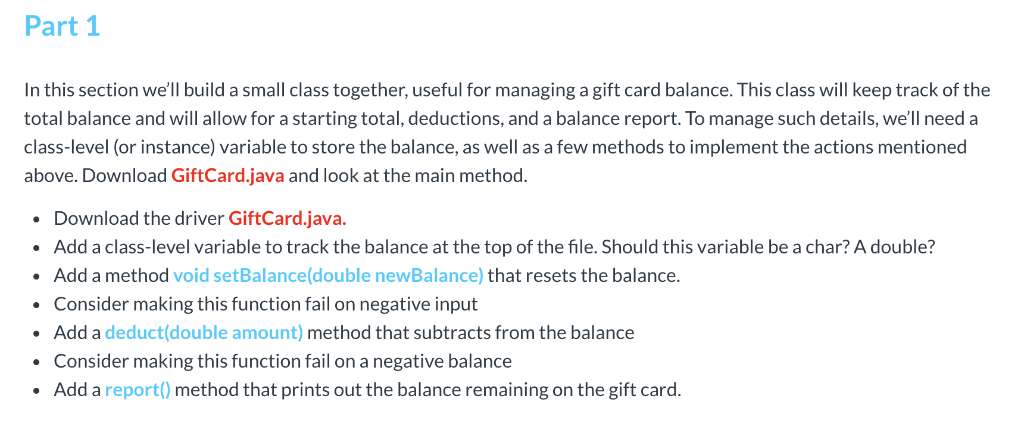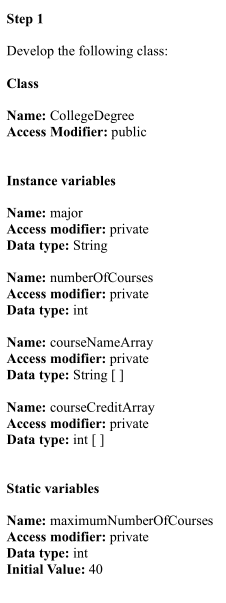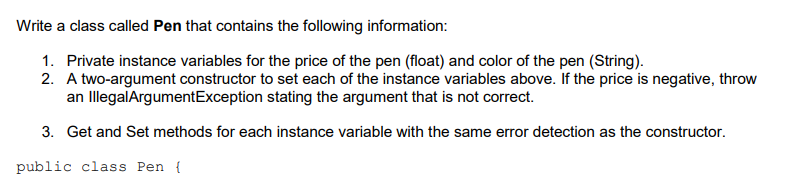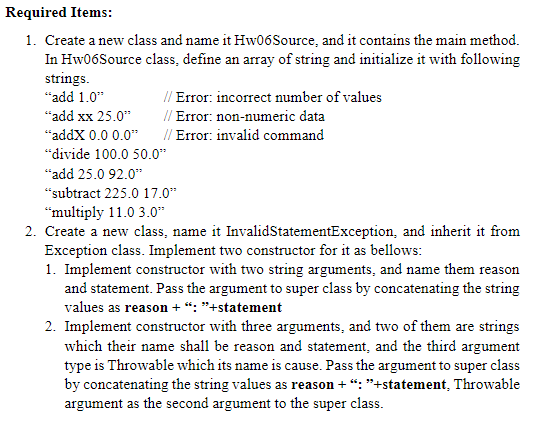# use java follow my code: public class q1 {    // Instance Variables    int currentFloor;...

use java

public class q1 {
// Instance Variables
int currentFloor;
double maximumWeight;
int topFloor;

// Constructor Declaration of Class
public q1 (int currentFloor, double maximumWeight, int topFloor)
{
this.currentFloor = currentFloor;
this.maximumWeight = maximumWeight;
this.topFloor = topFloor;
}

// Property to get value of currentFloor instance variable
public int getCurrentFloor()
{
return currentFloor;
}

// Property to get value of maximumWeight instance variable
public double getMaximumWeight()
{
return maximumWeight;
}

// Property to get value of topFloor instance variable
public int getTopFloor()
{
}
}

Instantiate a couple of

different Elevator

objects

Set their data members

Use Information Hiding

Labwork on Arrays Northwestern Polytechnic University Dr. Nels Vander Zanden 3

Write get/set methods

upOneFloor -- note cannot

exceed the top floor

downOneFloor – cannot go

below floor one

isAtTopFloor -- return true if

on the top floor, false

otherwise

public class q1 {
// Instance Variables
int currentFloor;
double maximumWeight;
int topFloor;

// Constructor Declaration of Class
public q1 (int currentFloor, double maximumWeight, int topFloor)
{
this.currentFloor = currentFloor;
this.maximumWeight = maximumWeight;
this.topFloor = topFloor;
}

// Copy constructor
public q1(Elevator el)
{
this.currentFloor = el.getCurrentFloor();
this.maximumWeight = el.getMaximumWeight();
this.topFloor = el.getTopFloor();
}

// Property to get value of currentFloor instance variable
public int getCurrentFloor()
{
return currentFloor;
}

// Property to get value of maximumWeight instance variable
public double getMaximumWeight()
{
return maximumWeight;
}

// Property to get value of topFloor instance variable
public int getTopFloor()
{
}

// Property to set the value of the currentFloor
public void setCurrentFloor(int currentFloor)
{
if(currentFloor >=1 && currentFloor <= top_floor)
this.currentFloor = currentFloor;
}

// Property to set the value of the maximumWeight
public void setMaximumWeight(double maximumWeight)
{
if(maximumWeight > 0)
this.maximumWeight = maximumWeight;
}

// Property to set the value of topFloor
public void setTopFloor(int topFloor)
{
if(topFloor > 1)
this.topFloor = topFloor;
}

// method to make the elevator go up by 1 floor, if possible
public void upOneFloor()
{
if(currentFloor < topFloor) // if not reached the top floor
currentFloor++;
}

// method to make the elevator go down by 1 floor, if possible
public void downOneFloor()
{
if(currentFloor > 1)
currentFloor--;
}

// method to check if the elevator is at top floor
public boolean isAtTopFloor()
{
return(currentFloor == currentFloor < topFloor);
}

public static void main(String[] args) {

Elevator ele1 = new Elevator(1,150,10); // create object using parameterized constructor
Elevator ele2 = new Elevator(ele1); // create object using copy constructor
// change the values of the data members
ele2.setCurrentFloor(3);
ele2.setMaximumWeight(120);
ele2.setTopFloor(5);

}

}

#### Earn Coin

Coins can be redeemed for fabulous gifts.

Similar Homework Help Questions
• ### Java template public class GiftCard { // Instance Variables // TODO: balance // Constructors /** *...Java template public class GiftCard { // Instance Variables // TODO: balance // Constructors /** * GiftCard(double initialBalance) * * Initialize a GiftCard object with a pre-defined amount of money. * * @param initialBalance double The desired starting balance */ public GiftCard(double initialBalance) { setBalance(initialBalance); } /** * GiftCard() * * Initialize a GiftCard object with a balance of \$0.00. */ public GiftCard() {} // Instance Methods /** * setBalance * * This should set the balance of the gift...

• ### Read through the code of the class Player, noting it has two instance variables, name and rank, which are of type String and three further instance variables won, drew and lost which are of type int....

Read through the code of the class Player, noting it has two instance variables, name and rank, which are of type String and three further instance variables won, drew and lost which are of type int. There is also an attribute of the class, points, which does not have a corresponding instance variable. Also note the constructor and methods of the class and what they do. TournamentAdmin class code: public class RankAdmin {    /**     * Constructor for objects of class...

• ### Create a class named GlobalWarming. In that class, define the following instance variables and methods:] Instance...

Create a class named GlobalWarming. In that class, define the following instance variables and methods:] Instance Variables name: A String that is the name of the person taking the quiz. Methods GlobalWarming () - A default or no argument constructor that takes no parameters and initializes the instance variable to ‘null’. GlobalWarming (String name) - A constructor that takes one parameter that will be the name of the person taking the quiz, and that will be used to initialize the...

• ### Step 1 Develop the following class: Class Name: CollegeDegree Access Modifier: public Instance variables Name: major...

Step 1 Develop the following class: Class Name: CollegeDegree Access Modifier: public Instance variables Name: major Access modifier: private Data type: String Name: numberOfCourses Access modifier: private Data type: int Name: courseNameArray Access modifier: private Data type: String [ ] Name: courseCreditArray Access modifier: private Data type: int [ ] Static variables Name: maximumNumberOfCourses Access modifier: private Data type: int Initial Value: 40 Constructor Name: CollegeDegree Access modifier: public Parameters: none (default constructor) Task: sets major to the empty string...

• ### Start a new project in NetBeans that defines a class Person with the following: Instance variables...

Start a new project in NetBeans that defines a class Person with the following: Instance variables firstName (type String), middleInitial (type char) and lastName (type String) (1) A no-parameter constructor with a call to the this constructor; and (2) a constructor with String, char, String parameters (assign the parameters directly to the instance variables in this constructor--see info regarding the elimination of set methods below) Accessor (get) methods for all three instance variables (no set methods are required which makes...

• ### Lab 7 Add three instance variables to your class RightTriangle from lab 5. The first two...

Lab 7 Add three instance variables to your class RightTriangle from lab 5. The first two are of type int and are called xLoc and yLoc and the third is of type int called ID. Also add a static variable of type double called scaleFactor. This should be initialized to a default value of 1. Update the constructor to set the three new instance variables and add appropriate get and set methods for the four new variables. All set methods...

• ### Programming assignment for Java: Do not add any other instance variables to any class, but you...Programming assignment for Java: Do not add any other instance variables to any class, but you can create local variables in a method to accomplish tasks. Do not create any methods other than the ones listed below. Step 1 Develop the following class: Class Name: College Degree Access Modifier: public Instance variables Name: major Access modifier: private Data type: String Name: numberOfCourses Access modifier: private Data type: int Name: courseNameArray Access modifier: private Data type: String Name: courseCreditArray Access modifier:...

• ### JAVA Write a class called Pen that contains the following information: 1. Private instance variables for...JAVA Write a class called Pen that contains the following information: 1. Private instance variables for the price of the pen (float) and color of the pen (String). 2. A two-argument constructor to set each of the instance variables above. If the price is negative, throw an IllegalArgumentException stating the argument that is not correct. 3. Get and Set methods for each instance variable with the same error detection as the constructor. public class Pen {

• ### In Java. Please use the provided code Homework 5 Code: public class Hw05Source { public static...In Java. Please use the provided code Homework 5 Code: public class Hw05Source { public static void main(String[] args) { String[] equations ={"Divide 100.0 50.0", "Add 25.0 92.0", "Subtract 225.0 17.0", "Multiply 11.0 3.0"}; CalculateHelper helper= new CalculateHelper(); for (int i = 0;i { helper.process(equations[i]); helper.calculate(); System.out.println(helper); } } } //========================================== public class MathEquation { double leftValue; double rightValue; double result; char opCode='a'; private MathEquation(){ } public MathEquation(char opCode) { this(); this.opCode = opCode; } public MathEquation(char opCode,double leftVal,double rightValue){...

Free Homework Help App